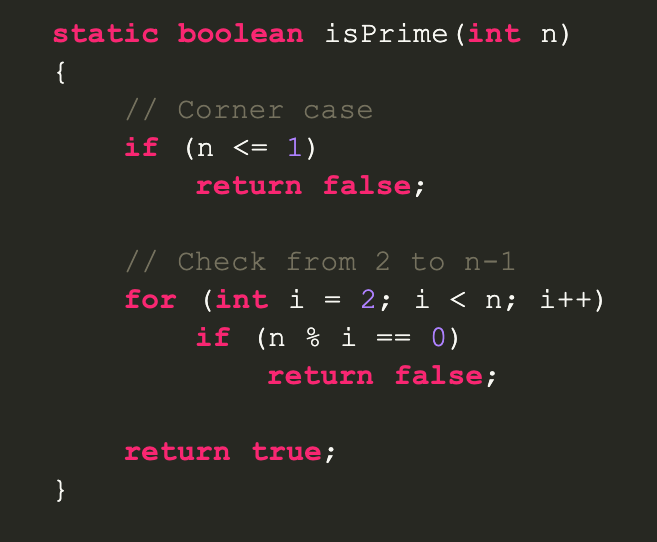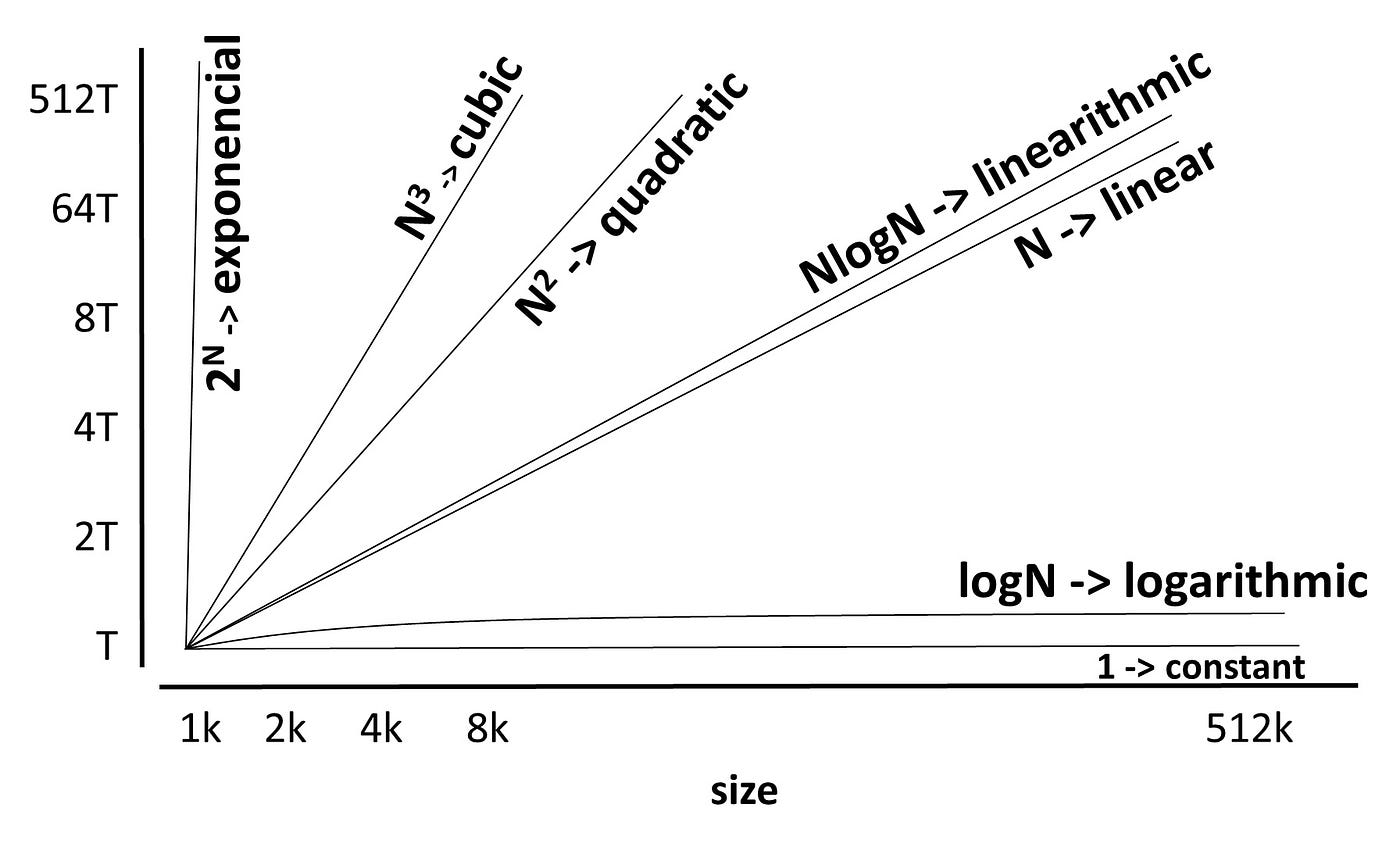# Big O Notation: Calculate Time & Space ComplexityIn order to check if a number is prime we will loop through all numbers from 2 to but excluding the actual number and check its divisibility.As an algorithm grows in size its execution time can be expected to increase. Unless in the case of constants, these usually involve simple calculations without loops.From the 1999 television-series “Big O”

# How to calculate Big O

To calculate Big O, there are five steps you should follow:

1. Break your algorithm/function into individual operations
2. Calculate the Big O of each operation
3. Add up the Big O of each operation together
4. Remove the constants
5. The highest term will be the Big O of the algorithm/function
`public class Add{  public static void main(String[] args)  {    int num1 = 8; // initialize num1 to be 8    int num2 = 4; // initialize num2 to be 4    System.out.println(num1 + num2); // Output should be 12  }}// Don't worry if you don't know Java syntax just know we are getting the sum of the two numbers`
`public class NestedLoop{  public static void main(String[] args)  {    int x = 0; // This will increment and serve as the outer counter    int y = 5; // This will decrement and serve as the inner counter        while (x < 10)    {      System.out.println("Outer counter: " + x);      while (y > 0)      {        System.out.println("Inner counter: " + y);        y = y - 1;      }      x = x + 1;    }    System.out.println("Loop Completed.");}`

# Summary

Big O notation can be hard to understand but it comes up very often in interviews and scaling sizable solutions. The mark of a great programmer is understanding the limits of their builds and improve them. Solving a problem can seem frustrating enough but it is important to think about this ideas of run time and space required.

--

--